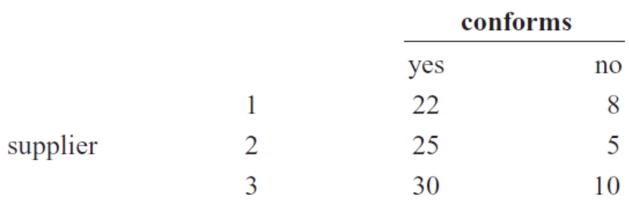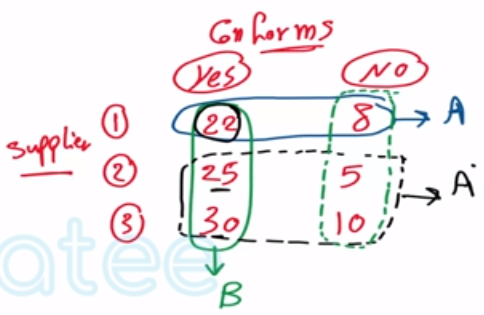Need Help?

Subscribe to Probability

###### \${selected_topic_name}
• Notes
• Comments & Questions

Samples of emissions from three suppliers are classi-
fied for conformance to air-quality specifications. The results
from 100 samples are summarized as follows:Let $A$ denote the event that a sample is from supplier 1 , and let
$B$ denote the event that a sample conforms to specifications.
Determine the number of samples in $A^{\prime} \cap B, B^{\prime},$ and $A \cup B$ .

$n=100$

$A \rightarrow$ from (1)

$B \rightarrow$ samples that conforms to specifications

$A^{\prime} \cap B$

$B^{\prime}, A \cup B$$A^{\prime} \cap B=25+30=55$

$B^{\prime}=8+5+10=23$

$A \cup B=8+22+25+30=85$

No comments yet